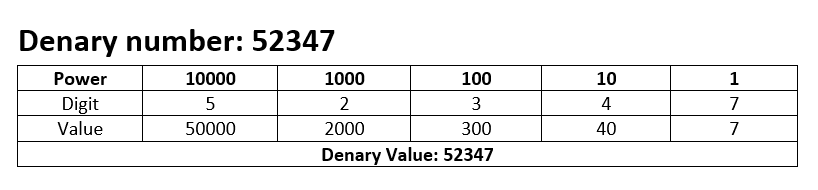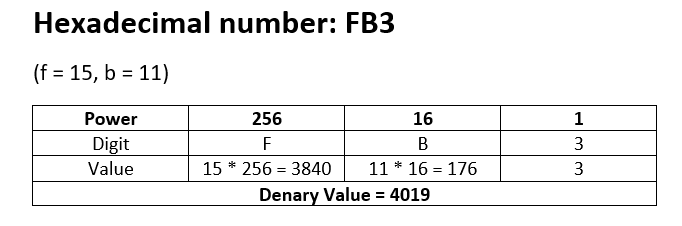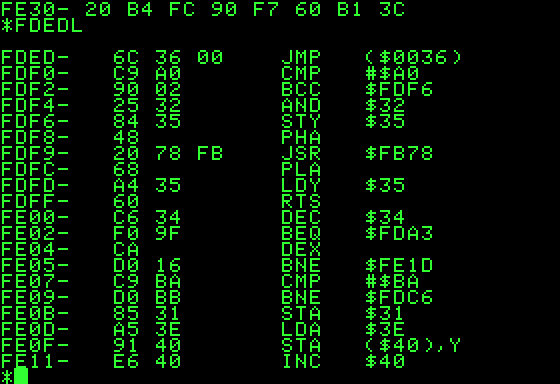## Introduction

### Why do we use it hexadecimal?

Binary is very difficult for humans to read (especially larger numbers) but computers work in binary, so any usage where you need to be able to inspect and edit the binary directly (such as in machine code/ assembly language) then humans need to be able to read the binary easily and accurately. This is where hexadecimal comes in :

• Hexadecimal allows humans to interact with binary in a human readable form.
• Large numbers can be represented in a smaller number of digits, making them easier and more accurately read by humans.
• Hexadecimal can be easily converted to binary and vice verse.

In the normal human counting system we use a base 10 number system, meaning that the each extra digit increases in powers of 10 each time:Hexadecimal is a Base 16 number system, meaning that increases in powers of 16, with each digit being one of 16 different combinations.

• 0 = 0
• 1 = 1
• 2 =2
• 3 = 3
• 4 = 4
• 5 = 5
• 6 = 6
• 7 =7
• 8 = 8
• 9 = 9
• A = 10
• B = 11
• C = 12
• D = 13
• E = 14
• F = 15

Why do we use a to f instead of 11 to 15?

We can’t use 11 to 15 because we wouldn’t know what number it represented. The Digits 13  could be 13 or it could be 19  (1 * 16 + 3), this is because denary numbers greater than 9 use 2 digits instead of one digit.## HTML/CSS

### Hexadecimal in HTML & CSS

Hexadecimal is used to represent RGB colors in HTML and in CSS. The video below shows how it works.

## MAC

MAC addresses are unique identifier codes given to each piece of network enable hardware created. They are issued by the manufacturer of the hardware when the device is created and the first part of the MAC address indicates the manuafacturer.

MAC addresses consist of 6 pairs of hexadecimal digits, separated by a colon.

Example: dd:f2:c2:a1:2b:cd

Each pair can be one of 256 possible values (0-255) so the total number of possible MAC addresses are 256 to the power of 6 = 281,474,976,710,656 possible MAC addresses.

### Why are MAC addresses displayed in HEX?

MAC addresses are displayed in hex a because the codes are too long for humans to be able to read accurately in binary.

The MAC address above in binary would be 110111011111001011000010101000010010101111001101

This is incredibly difficult for a human to read/remember.

## Assembly/MC

### Assembly Language & Machine Code

Hexadecimal is used when humans are reading/editing machine code or assembly language. Computers can only interpret instructions written in binary, so all instructions need to be written in binary. Humans would find this incredibly slow and difficult to do so we display the hexadecimal equivalent to humans to read. This means that humans can edit machine code.Source: Wikipedia